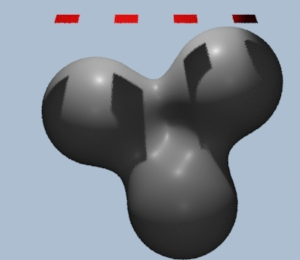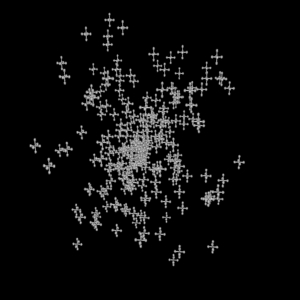### RfMExample Python Rifs I

#### Introduction

The python scripts presented here are intended to illustrate some of the tasks that Rifs can perform. The reader should review the tutorial RfM: Batch Filtering before continuing with this tutorial.

#### Using the Rifs with Maya

Using the batchRenderRI() proc with Maya (see RfM: Batch Rendering) a Rif or Rifs may be specified as follows.

```    batchRenderRI("rif_it", 1);
or
batchRenderRI("rif_it.Rif(), 1");```

The second arg indicates whether "rman genrib" should be used to generate a fresh set of ribs. If only the name of module is specified then ribops.py assumes the name of the class is 'Rif'. Therefore, `rif_it` is considered to be the same as `rif_it.Rif()`. Multiple Rifs can be specified as follows.

`    batchRenderRI("rif_it;rif_meshToBlobby(0.5)");`

Note the use of a semi-colon to separate the names of the Rifs. Spaces must not appear anywhere within the double quotations. String arguments must be specified with single quotes ie.

`    batchRenderRI("rif_it;rif_shadinginterpolation('smooth'), 1");`

#### Example 1: Saving a Deep Image (.dtex)

The first Rif edits the Display statement of a rib so that a deep image file is saved to disk - suitable for deep compositing in Nuke. For example,

`    batchRenderRI("rif_deepimage.Rif(), 1");`

would convert a Display statement such as,

```    Display "renderman/first/images/first.0003.iff" "mayaiff" "rgba"
to
Display "renderman/first/images/first.0003.dtex" "deepshad" "rgba"```

Listing 1 (rif_deepimage.py)

 ```import prman, os class Rif(prman.Rif): def Display(self, name, driver, channels, params): if driver != 'shadow' and driver != 'deepshad' and driver != 'null': driver = 'deepshad' name = os.path.splitext(name) name = name + '.dtex' self.m_ri.Display(name, driver, channels) else: self.m_ri.Display(name, driver, channels, params)```

#### Example 2: Converting a Standard Blobby to a Volume

The third Rif converts a "standard" blobby produced by, say, a Maya particle emitter to a blobby that can be rendered as a volume primitive. A typical Maya/RfM rib archive that defines a standard Blobby primitive will look like this,

```##RenderMan RIB
version 3.04
Blobby 3
[1001 0
1001 16
1001 32
0 3   0 1 2]
[1 0 0 0  0 1 0 0  0 0 1 0 -0.5  0.2  0.0 1
1 0 0 0  0 1 0 0  0 0 1 0  0.9  0.5  0.0 1
1 0 0 0  0 1 0 0  0 0 1 0  0.5 -0.5  0.0 1]
[""]```

When rendered the Blobby would appear as shown in figure 2. But when converted to a volume primitive and rendered with an appropriate surface shader it will look completely different - figure 3.Figure 2 A standard BlobbyFigure 3 A Volume Blobby

A Blobby can be rendered as a volume primitive if its sequence of opcodes begin with 8.

```    Blobby 3
[8
1001 0
1001 16
1001 32
0 3   0 1 2] ...transformation data...```

Currently RMS (version 3) does not provide a convenient way of specifying a Blobby as a volume primitive. The Rif shown in listing 3 adds the required opcode.

Listing 3 (rif_volumeblobby.py)

 ```import prman class Rif(prman.Rif): def Blobby(self, numblobs, opcodes, xyz, strs, params): opcodes = (8,) + opcodes self.m_ri.Blobby(numblobs, opcodes, xyz, strs, params) ```

#### Example 3: Converting a Polymesh to a Blobby

The next Rif converts all polymeshes, specified in a rib by the `PointsGeneralPolygons` statement, to a `Blobby`. Figure 4 shows the appearance of a mesh in Maya and how it might appear in the final image.

Listing 4 (rif_meshToBlobby.py)

 ```import prman class Rif(prman.Rif): def __init__(self, ri, scale): self.scale = float(scale) prman.Rif.__init__(self, ri) def PointsGeneralPolygons(self, nloops, nverts, verts, params): opcodes = [] numblobs = len(params['P'])/3 for n in range(numblobs): opcodes.append(1001) opcodes.append(n * 16) opcodes.append(0) # blending code opcodes.append(numblobs)# blend all blobs for n in range(numblobs): opcodes.append(n) # indices of the blobs to blend common = (self.scale,0,0,0,0,self.scale,0,0,0,0,self.scale,0) transforms = (self.scale,0,0,0,0,self.scale,0,0,0,0,self.scale,0) xyz = params['P'] numxyz = len(xyz) for n in range(0, numxyz, 3): pos = (xyz[n], xyz[n+1], xyz[n+2]) if n == 0: transforms = common + pos + (1,) else: transforms = transforms + common + pos + (1,) params = {} strs = ('',) self.m_ri.Blobby(numblobs,opcodes,transforms, strs, params)```Figure 4
PointsGeneralPolygons converted to a Blobby

#### Example 4: Changing the Width of Points

This Rif changes the diameter (constantwidth) of the points defined by the rib `Points` statement. Notice in this example the Points() method receives two inputs, num_points and params, but the call to the base class, self.m_ri.Points(params), outputs only one argument.

Listing 5 (rif_points_width.py)

 ```import prman class Rif(prman.Rif): def __init__(self, ri, width): self.width = (width,) prman.Rif.__init__(self, ri) def Points(self, num_points, params): # two args in params['constantwidth'] = self.width self.m_ri.Points(params) # one arg out```

#### Example 5: Substituting Archives for Points

This Rif reads the xyz locations of the points defined by the rib `Points` statement and outputs an archive at each location.

Listing 6 (rif_points_archive.py)

 ```import prman class Rif(prman.Rif): def __init__(self, ri, archive_path): self.archive_path = archive_path prman.Rif.__init__(self, ri) def Points(self, num_points, params): if self.archive_path != '': coords = params['P'] for n in range(0, num_points, 3): x = coords[n] y = coords[n+1] z = coords[n+2] self.m_ri.TransformBegin() self.m_ri.Translate(x,y,z) self.m_ri.ReadArchive(self.archive_path) self.m_ri.TransformEnd() else: self.m_ri.Points(params)```Figure 5 Regular PointsFigure 6 Points replaced by archives

#### Example 6: Changing the Size (Scale) of a Blobby

This Rif receives a tuple of transformation values, 16 per blob, in the xyz argument. For ease of handling, the rif converts the tuple to a list and then divides it into a list of sublists - each sublist containing the 16 transformation values for a blob. Each of three entries, the transformation scaling factors, are assigned a new scaling value. Finally, using the itertools.chain() function the list of edited sublists are converted to a single tuple that is passed to the output.

Listing 6 (rif_blobby.py)

 ```# A rif that uniformly changes the size (scale) of each # blob in a blobby. # M.Kesson # March 1 2018 import prman import itertools class Rif(prman.Rif): def __init__(self, ri, scale): prman.Rif.__init__(self, ri) self.scale = scale def Blobby(self, numblobs, opcodes, xyz, strs, params): # Chop up the single tuple of coordinates into lists that # contain the 16 (matrix) transformation values for each blob. matrices = [ list(xyz)[offs:offs + 16] for offs in range(0, len(xyz), 16) ] xyz = () # Now we have the data in lists we can alter their values for matrix in matrices: matrix = self.scale matrix = self.scale matrix = self.scale # Convert the list of lists back to a single tuple. xyz = tuple(itertools.chain(*matrices)) self.m_ri.Blobby(numblobs, opcodes, xyz, strs, params) ```

© 2002- Malcolm Kesson. All rights reserved.